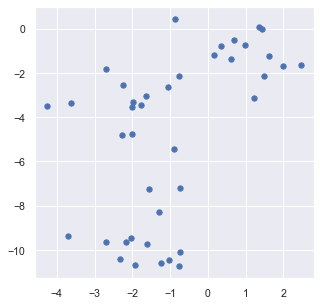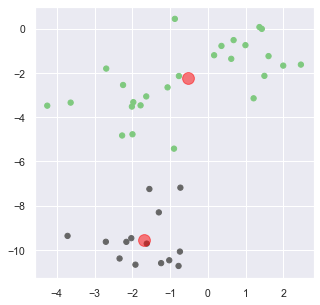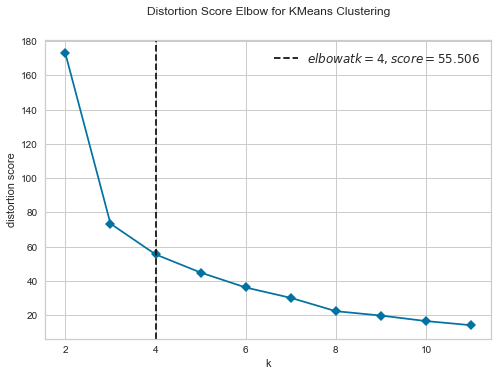# Notebook 2.4 - Clustering with sklearn¶

In :
import matplotlib
import matplotlib.pyplot as plt
import seaborn as sns; sns.set()


We begin with some housekeeping. We will be using the matplotlib and seaborn packages to plot the charts in this notebook.

In :
plot_size   = 14
plot_width  = 5
plot_height = 5

params = {'legend.fontsize': 'large',
'figure.figsize': (plot_width,plot_height),
'axes.labelsize': plot_size,
'axes.titlesize': plot_size,
'xtick.labelsize': plot_size*0.75,
'ytick.labelsize': plot_size*0.75,
plt.rcParams.update(params)


As we are also using the outputs from the charts to create the illustrations in our lecture slides, we require an additional level of control over the appearance of the charts.

The above commands are therefore used to override the default font and any dimension parameters.

In :
from sklearn.cluster import KMeans
from sklearn.datasets import make_blobs


We use the KMeans algorithm implementation from the sklearn package. sklearn also provides several other clustering algorithms under the sklearn.cluster namespace. You can read more about the other algorithms here.

Also from sklearn, we are using the make_blobs command to create a random dataset. As the command name implies, it creates a vertex arrangements that is concentrated around a defined number of "blobs".

In :
num_customers = 40

In :
coord, clust_true = make_blobs(n_samples=num_customers,
centers=3,
cluster_std=1,
random_state = 2)


The make_blobs command returns two outputs, which we save on the coord and clust_true variables. Let's see what the contain.

In terms of input parameters, aside from the number of elements that we want to create, we can also define how many blobs we are using (centers) and also how far away will the samples be from each "blob" centroid.

As the outputs are random, we are using random_state to provide a "seed" to the underlying random number generator used by the function, which will ensure that we get the same results, every time.

In :
coord

Out:
array([[ -1.54915892,  -7.25010857],
[  0.61758013,  -1.36802291],
[  1.36369409,   0.06608172],
[  0.35857025,  -0.7851559 ],
[ -0.89752435,  -5.42677013],
[ -3.71486953,  -9.36874886],
[ -4.25209341,  -3.4847562 ],
[  1.60459034,  -1.24558156],
[ -0.8748411 ,   0.43763252],
[ -1.99653623,  -4.77782225],
[  1.20936556,  -3.15216453],
[ -1.23856256, -10.59940081],
[ -2.02797291,  -9.47245011],
[ -0.74104364, -10.07763506],
[ -0.77722054, -10.72676345],
[ -1.91775697, -10.66908765],
[ -2.27031954,  -4.83274261],
[ -0.72864791,  -7.18926735],
[ -3.63296701,  -3.34704806],
[  0.67974136,  -0.52254041],
[ -1.97416044,  -3.32681457],
[ -1.61892392,  -9.71765939],
[ -0.76794095,  -2.14509066],
[ -2.15820985,  -9.63790953],
[ -1.29923245,  -8.30647414],
[ -2.01196044,  -3.52563248],
[ -2.33805418, -10.39048298],
[ -1.06834753,  -2.658024  ],
[  1.49510676,  -2.13776585],
[  1.42674589,  -0.01517292],
[  1.99361544,  -1.67464467],
[ -2.70131918,  -9.63497056],
[ -1.02353151, -10.47025441],
[ -1.6322142 ,  -3.06730015],
[  2.46092757,  -1.62922949],
[ -1.78211322,  -3.47052225],
[ -2.69138291,  -1.80881652],
[  0.16411427,  -1.20584193],
[  0.99325932,  -0.75119958],
[ -2.24589423,  -2.5508473 ]])
In :
clust_true

Out:
array([0, 1, 1, 1, 2, 0, 2, 1, 1, 2, 1, 0, 0, 0, 0, 0, 2, 0, 2, 1, 2, 0,
2, 0, 0, 2, 0, 2, 1, 1, 1, 0, 0, 2, 1, 2, 2, 1, 1, 2])

It appears that coord is a two-dimensional array of X,Y coordinates. clust_true returns the index of the cluster that make_blobs things that each coordinate should belong to.

It would be interesting to refer to this array later on, but we are not going to be using it for the purposed of our analysis. We will determine the clusters on our own, with the help of the KMeans algorithm.

In :
plt.scatter(coord[:, 0],
coord[:, 1],
s=plot_size*2,
cmap='viridis');We are using the scatter command to plot the customer locations. The s parameter defines the size nodes, while the cmap parameters picks a color scheme from the seaborn library.

#### Why don't you experiment with different values for centers and cluster_std?¶

In :
model = KMeans(n_clusters=2)

model.fit(coord)

clust_pred = model.predict(coord)


Running the algorithm involves 3 distinct steps:

1) We initialise a KMeans instance, and decide how many clusters we are going to seek (here: 2)

2) We "train" the model using the coordinates using the fit() function.

3) using the predict() function, calculate the centroids.

In :
plt.scatter(coord[:, 0],
coord[:, 1],
c = clust_pred,
s=plot_size*2,
cmap='Accent')

centers = model.cluster_centers_

plt.scatter(centers[:, 0],
centers[:, 1],
c = 'red',
s=plot_size*10,
alpha=0.5);We are using the scatter command twice. The first time, we use it to plot the customer coordinates.

Instead of a color, we supply to c the predicted index of the cluster. The Accent color scheme, will automatically transform this to a visually appealing (hopefully) combination of colours.

The second time that we use scatter we add the centroids of our customers to the original graph.

As a rule of thumb, every additional matplotlib command within a Jupyter cell will simply update the previous plot that was created within the cell.

In :
model.inertia_

Out:
172.85989892525026

We can obtain the inertia of our model (within-cluster sum of squares) from the .inertia_ field, which provides us a measure of how well the KMeans has performed

In :
from yellowbrick.cluster import KElbowVisualizer

visualizer = KElbowVisualizer(model, k=(2,12),timings=False)
visualizer.fit(coord)   # Fit the data to the visualizer
visualizer.show()       # Finalize and render the figure

/Users/pan/anaconda3/lib/python3.7/site-packages/sklearn/utils/deprecation.py:143: FutureWarning: The sklearn.metrics.classification module is  deprecated in version 0.22 and will be removed in version 0.24. The corresponding classes / functions should instead be imported from sklearn.metrics. Anything that cannot be imported from sklearn.metrics is now part of the private API.
warnings.warn(message, FutureWarning)Out:
<matplotlib.axes._subplots.AxesSubplot at 0x7fc56b004c90>

As discussed in the class, we are using the elbow method to automatically identify a suggested number of clusters, without supplying any additional contextual information about the problem.

This technique usually works well as a preliminary step while performing an initial shift through data. It must, however, be followed up with a proper facility location analysis.

In this case we are using the KElbowVisualizer that is provided by the yellowbrick package. With the parameter k we are instructing the algorithm to check all values between 2 and 12 clusters.

The term "distortion" refers to the within-cluster sum of squares difference between all elements and their corresponding centroids, and is therefore equivalent to "inertia"